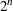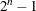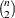The HPSUMMARY Procedure

How PROC HPSUMMARY Groups Data

Groups of observations are defined by specifying certain variables as classification variables in the CLASS statement. Unique values of the n CLASS variables are used to partition the input data, and the resulting summarized data (one observation per group) is called the "n-way."

PROC HPSUMMARY can also combine the partitioned groups into larger groups by removing one or more CLASS variables from consideration when grouping. There aredifferent groupings that can be generated from n CLASS variables. Each of these groupings is a "type," which appears in the output data set as a variable named _TYPE_. Type 0 includes no CLASS variables and summarizes the entire input data set, Type 1 includes only the last CLASS variable specified, and so on to Type, which is the n-way.

By default, PROC HPSUMMARY generates only the n-way. The option ALLTYPES (or ALLWAYS) in the PROC HPSUMMARY statement generates alltypes. You can also use either of the following statements to choose which types appear in the output data set:

• The WAYS statement specifies how many CLASS variables appear in each output type. For example, WAYS 1 produces types for each CLASS variable individually, WAYS 2 generates allpossible pairs, and so on.

• The TYPES statement explicitly specifies the desired types by CLASS variable name, such as TYPES A A*B C (), where A*B might specify Type 6 and "()" specifies Type 0.

The TYPES statement controls which of the available classification variables PROC HPSUMMARY uses to subgroup the data. The unique combinations of these active classification variable values that occur together in any single observation of the input data set determine the data subgroups. Each subgroup that PROC HPSUMMARY generates for a given type is called a level of that type. For all types, the inactive classification variables can still affect the total observation count of the rejection of observations with missing values. When you use a WAYS statement, PROC HPSUMMARY generates types that correspond to every possible unique combination of n classification variables chosen from the complete set of classification variables. For example

```proc hpsummary;
class a b c d e;
ways 2 3;
output out=results;
run;
```

is equivalent to

```proc hpsummary;
class a b c d e;
types a*b a*c a*d a*e b*c b*d b*e c*d c*e d*e
a*b*c a*b*d a*b*e a*c*d a*c*e a*d*e
b*c*d b*c*e c*d*e;
output out=results;
run;
```

If you omit the TYPES statement and the WAYS statement, then PROC HPSUMMARY uses all classification variables to subgroup the data (the NWAY type) for the output data set.